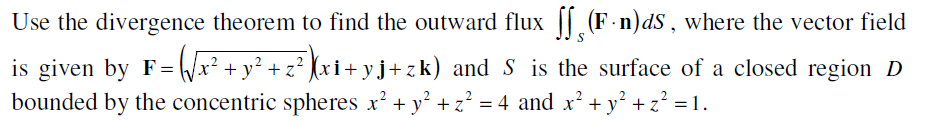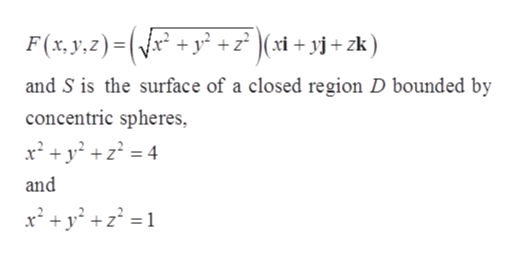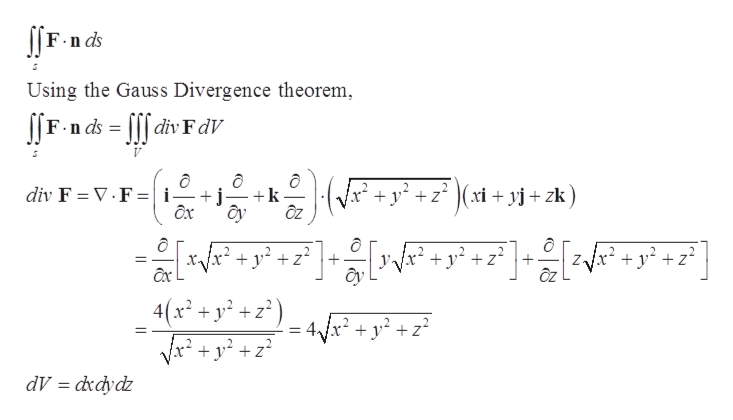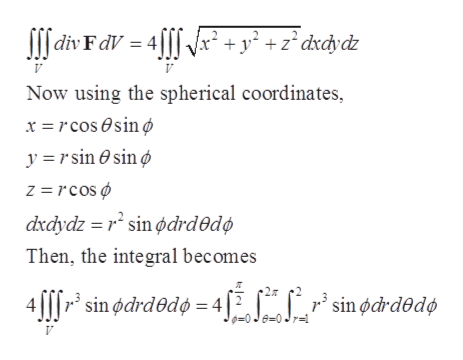# Use the divergence theorem to find the outward flux || (F-n)dS, where the vector fieldis given by F= /x² + y² + z² (xi+ yj+zk) and S is the surface of a closed region Dbounded by the concentric spheres x' + y' + z² = 4 and x' + y° +z? = 1.

Question
6 viewshelp_outlineImage TranscriptioncloseUse the divergence theorem to find the outward flux || (F-n)dS, where the vector field is given by F= /x² + y² + z² (xi+ yj+zk) and S is the surface of a closed region D bounded by the concentric spheres x' + y' + z² = 4 and x' + y° +z? = 1. fullscreen
check_circle

star
star
star
star
star
1 Rating
Step 1

Given that the vector field,help_outlineImage TranscriptioncloseF (x, y,z)=(x + y² + z° )(xi + yj + zk) and S is the surface of a closed region D bounded by concentric spheres, x² + y? + z? = 4 and x² +y* +z° =1 fullscreen
Step 2

Using the formula of outward flux of vector field,help_outlineImage TranscriptioncloseJ[F-n ds Using the Gauss Divergence theorem, |[F-n ds = [[[ div FdV (Vx² + y² + z² )(xi + yj + zk) div F = V.F =|i- + j- +k Ôx *Vx* + y? +z° +- + y? +z? + y? +z? y. ây 4(x² + y² + z² ) Vx? + y? + z? 4x² + y² + z? dV = dxdydz fullscreen
Step 3

Then, the integra...help_outlineImage TranscriptioncloseSI[ div FdV = 4f[[ \x* + y² +z°dxcydz Now using the spherical coordinates, x = r cos esin Ø y = r sin O sin ø z = rcos Ø dxdydz = r* sin ødrd®dø Then, the integral becomes 27 4|||r* sin ødrdedø = 4|2 sin ødrdedø 0=0 Je=0 Jr= fullscreen

### Want to see the full answer?

See Solution

#### Want to see this answer and more?

Solutions are written by subject experts who are available 24/7. Questions are typically answered within 1 hour.*

See Solution
*Response times may vary by subject and question.
Tagged in

### Math